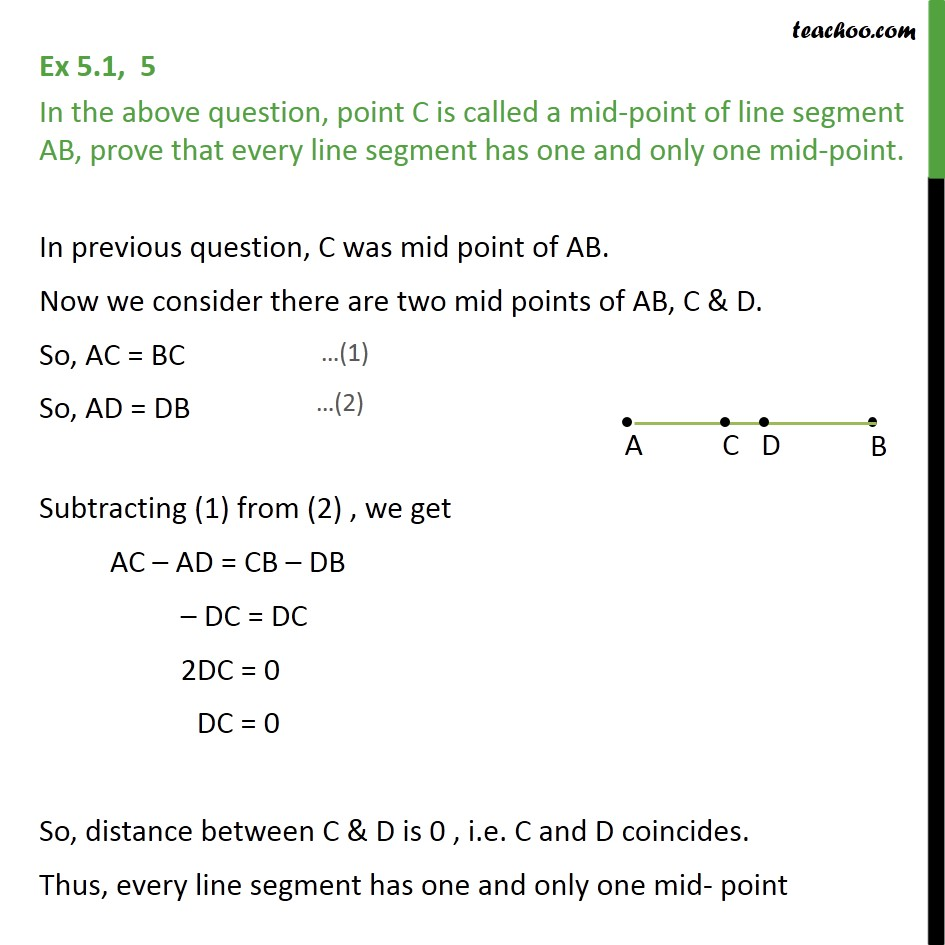Ex 5.1

Chapter 5 Class 9 Introduction to Euclid's Geometry
Serial order wiseSolve all your doubts with Teachoo Black (new monthly pack available now!)

### Transcript

Ex 5.1, 5 In the above question, point C is called a mid-point of line segment AB, prove that every line segment has one and only one mid-point. In previous question, C was mid point of AB. Now we consider there are two mid points of AB, C & D. So, AC = BC So, AD = DB Subtracting (1) from (2) , we get AC – AD = CB – DB – DC = DC 2DC = 0 DC = 0 So, distance between C & D is 0 , i.e. C and D coincides. Thus, every line segment has one and only one mid- point